KNOWLEDGEBASE - ARTICLE #2229

# Bug Fixed: Asymmetrical Confidence Intervals for Probability of Death (Kaplan-Meier Survival Analysis) reported incorrectly prior to Prism 9.4.1

### The big picture

With the release of Prism 9.4.1, an issue was fixed in which some results were reported incorrectly for the Kaplan-Meier Survival Analysis in Prism using a particular set of options. Specifically, this issue was only observed when the analysis was set to report the Probability of Death and to report the asymmetrical 95% confidence intervals of these probabilities. By default, Prism reports the Probability of Survival and does not report confidence intervals for this analysis, and so the issue would only be observed by specifying these options.

### The details

When performing Kaplan-Meier Survival Analysis, Prism provides the option to specify how the primary results should be reported: as the Probability of Survival (default and more common) or the Probability of Death (less common, but mathematically equivalent). The Probability (proportion or percentage) of Death is calculated as the complement to the corresponding Probability of Survival:

Death (Percentage) = 100 - Survival (Percentage),
Death (Proportion) = 1 - Survival (Proportion)

In Prism prior to version 9.4.1, both the Probability of Survival and the Probability of Death (reported as a percentage or proportion) were correct. Additionally, if (asymmetrical) 95% confidence intervals were specified to be reported, these were also correctly reported for the Probability of Survival. However, the method for calculating the (asymmetrical) 95% confidence interval for the Probability of Death was incorrect.

Results in versions prior to Prism 9.4.1

 Survival (Percent): +Error and -Error Correct Death (Percent): +Error and -Error Incorrect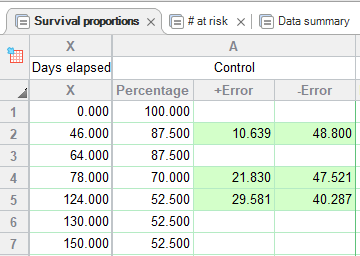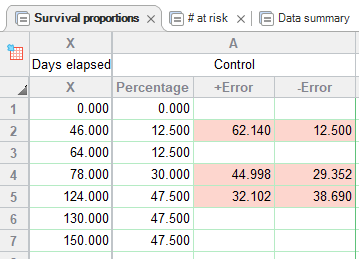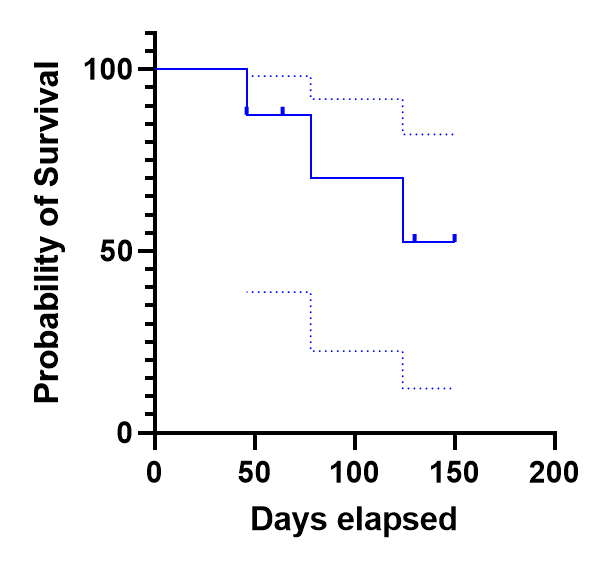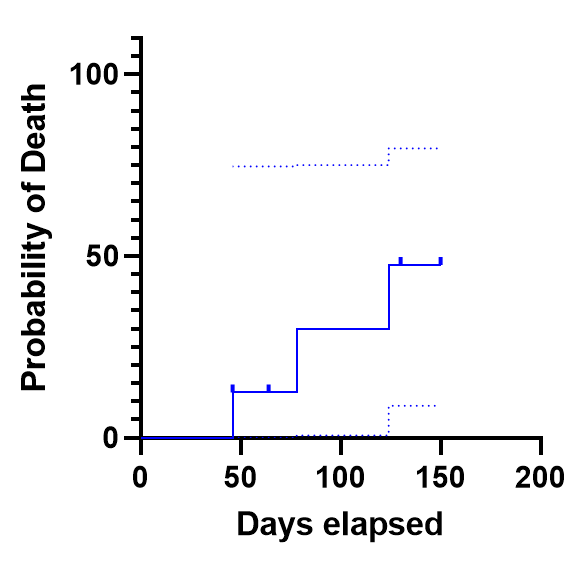Updated results beginning in version 9.4.1

 Survival (Percent): +Error and -Error Correct Death (Percent): +Error and -Error Incorrect Same as in previous versions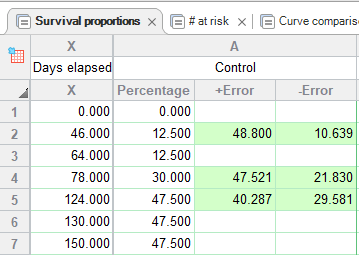Same as in previous versions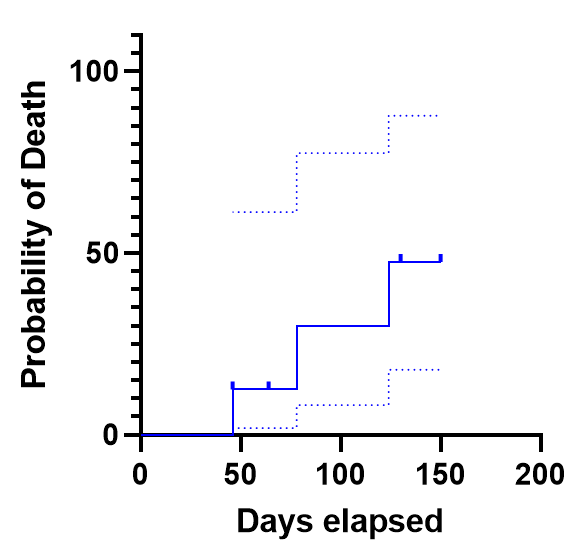### Obtaining correct 95% confidence intervals in Prism versions prior to 9.4.1

Because of the relationship between the Probability of Survival and the Probability of Death (see above), it is possible to calculate the correct values for the 95% confidence interval for the Probability of Death from the values for the 95% confidence interval for the Probability of Survival. In fact, the conversion is quite simple, and relies on understanding the relationship between the +Error and -Error for the Survival and Death curves:

+Error (Death) = -Error (Survival)
-Error (Death) = +Error (Survival)

Keywords: Survival analysis bug Probability of Death

## Explore the Knowledgebase

Analyze, graph and present your scientific work easily with GraphPad Prism. No coding required.